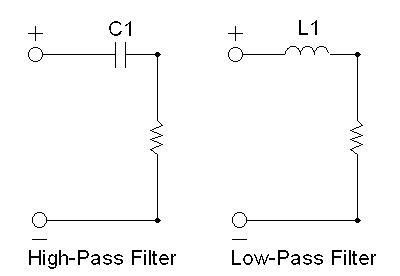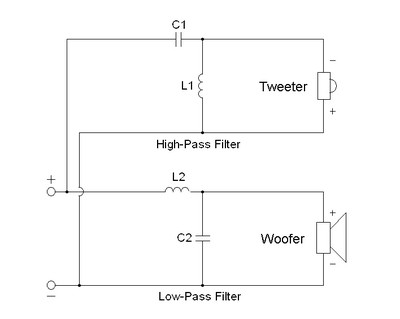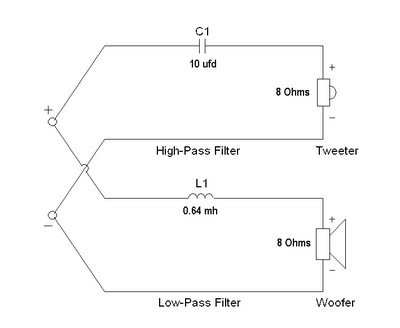“Let our rigorous testing and reviews be your guidelines to A/V equipment – not marketing slogans”

# The Crossover - Brain of your Loudspeaker System

by June 09, 2009
Contributors:

Have you ever wondered how the tweeter and woofer have their responses combined in a speaker system, or what the crossover network is and how it works? If so, this article will shed some light on the least seen and perhaps most undervalued part of the speaker system, the crossover network. Unlike the electronic world where the filters have gone from using tubes, to analog computers to digital signal processors, modern day passive crossovers, a pair of frequency selective filters designed to work together as a single network, are basically the same as they were about 100 years ago when the speaker business was in its infancy. While crossover components and materials and construction have changed, the underlying theory and practice has not.

### What does the Crossover Do?

Like your own brain, the crossover network is a director. In addition to its primary function, the passive crossover in practice is expected to do more than simply split the frequency band in two.

1) The crossover allows you to place two 8 ohm speakers in parallel, one for the highs and one for the lows, and still present an 8 ohm, not a 4 ohm load to the amplifier (which is what the amp would see without the network)

2) The crossover allows you to match two drive units of different efficiencies so that the combination of the two does not result in a large shelf in the frequency response.

3) The crossover allows you to equalize the frequency response of either the woofer or tweeter, making the composite response smoother than the unfiltered curves of either driver (woofer or tweeter) would seem to indicate is possible.

Let’s get back to the basic job of the crossover, and see if we can understand how it accomplishes this by learning a few new but simple concepts. The basic job is to send the high frequency information to the tweeter, and eliminate this same band of high frequencies from the woofer. Next, send the low frequency information to the woofer, and eliminate this same band of low frequencies from the tweeter. A crossover is in essence two frequency filter sections working in parallel. A high pass section and a low pass section. These terms are self explanatory. The high pass passes high frequencies, the low pass passes low frequencies. The high pass is placed in series with the tweeter, and the low pass is in series with the woofer. A normal two way crossover will have six terminals. Two in, and four out. Usually the two sections (high-pass and low-pass) are in parallel and both sections ONLY work properly when the woofer and tweeter are both attached and operational. To understand how the crossover does its job, we must first understand the concept of impedance.

The three main passive elements used in all crossovers are resistors, inductors, and capacitors. Impedance is a measure of how much the resistor, capacitor or inductor impedes the flow of electrons at any given frequency. Resistors are the simplest components, and the easiest to understand. The have a constant resistance to the flow of electrons which does not vary with the frequency of the signal. Resistors do not change the phase of the signal, the time relationship between voltage (electrical pressure) and current (flow of electrons). They only consume power and convert it to heat. That is all they do. In a perfect speaker system there is no need for resistors at all. Of course, while there are no perfect speakers, all the basic crossover theory's we use start with that premise.

The second element we shall consider is a capacitor. The capacitor impedes the flow of electrons through it in a way which is NOT independent of frequency. (It would not be technically correct to use the word resistance, but you can certainly think of it as such to conceptualize this). The capacitor has an impedance which is inversely related to frequency. This is to say when you double the frequency of a signal applied to the capacitors terminals, its impedes that electrical flow by half as much as it did at the lower frequency. If you halve the frequency applied, the capacitors impedance (How much it impedes the flow of electrons) is doubled. This is a very useful characteristic which we use to create networks which are frequency selective (like a crossover).

### The Maths

The impedance of a capacitor is determined by the equation: Z = 1/j(2piF*C)

Where Z = Impedance magnitude,

J = the square root of (-1) ("Imaginary" component to describe the phase)

pi = 3.1414 etc

C = Value of Capacitance in Farads; 1 Farad = 1,000,000 ufd

I think the first time I read about the square root of (-1) I had an allergic reaction. So, for those of us who are finding it difficult to comprehend phase for the first time, we can pretend it is not there, and to simplify the discussion we will admit to this equation being somewhat incomplete without the “j”, but for the determination of the steady state and not transient value of the impedance, we are OK.

When a capacitor is put in series (shares one electrical terminal) with a resistor, the capacitor acts as a high pass filter. At some frequency it has the same impedance as the resistor, and at a much higher frequency it is like a short, just a piece of wire with little to no resistance (at least in a perfect capacitor). At very low frequencies the impedance of the capacitor becomes so high, that essentially nothing can pass through it. It is this characteristic which is used in endless electronic circuits to allow AC signals to pass through yet completely block DC voltages from one part of the circuit to another.Figure 1

Lets say we have a 10 ufd capacitor and put in in series with an 8 ohm resistor. At some frequency, the capacitors impedance magnitude will be equal to the 8 ohm impedance of the resistor. (Which of course is still 8 ohms at any frequency from DC to daylight.)

Lets do the math.

Z = 8 = 1/{(2)*(3.14)*(F)*(10)*(10-6)}

The capacitor is MICRO Farads, which is millionths of a Farad, so we must multiply the 10 by 0.000001( 10-6) to convert microfarads into Farads, so we can solve for the frequency (F) in Hz. By cross multiplying (multiplying both sides by F and dividing both sides by 8) we get:

F = 1/(2*3.14*8*10*10-6)) = 1990 Hz.

What this tells us is that at 1990 Hz (2 kHz if you are among friends) the impedance of the capacitor is equal to that of the resistor, so at this point the power into the resistor is half what it would be without the capacitor. Put another way, at 1990 Hz, the power into the resistor is 3 db less and this is your crossover point. Above this frequency the attenuation (reduction in power to the resistor) is less, and as we go lower in frequency the capacitors impedance grows larger, while the resistor is unchanged, so the attenuation of signal to the resistor increases. At a frequency of 995 Hz, the attenuation will be 6 db. At 498 Hz, it will be 12 db. We call this a first order or 6 db per octave crossover. That is, in a nutshell, how this works. It grows in complexity when more parts are used, but the basic function is the same. Now if your speaker is a perfectly resistive load, your 10 ufd series capacitor will be a perfect passive 6 db per octave High Pass (HP) filter. Don't worry, life is not that simple.

The other important element used in a crossover network is the Inductor; sometimes called a choke, and sometimes called a coil. They are all refer to the same thing. The Inductor is in many ways the inverse of the capacitor. It too changes impedance with frequency, but in a way entirely opposite to the capacitor. The inductors impedance grows LARGER with increasing frequency, not smaller like the capacitor. Its impedance grows larger at the same rate as the capacitor grows smaller, and the similarity ends there. So if we replace our capacitor with an inductor in the first case we have a first order low pass filter. (As shown in figure # 1)

Note that in higher order networks, second order and above, both inductors and capacitors are used in the same circuit to provide high-pass (HP) or low-pass (LP) filtering. In the HP filter, capacitors are put in series with the load while inductors are put in parallel. (A resistor represents the load in figure 1, and the speakers represent the load in figure 2). In the LP filter the opposite is true, the inductors are in series and the capacitors in parallel with the load (or speaker if you are making a crossover network). Hopefully this makes sense if you keep in mind the direction of the impedance changes of the parts with increasing or decreasing frequency.Figure 2

In the network pictured in figure 2, the HP blocking capacitor, C1, increases in impedance with decreasing frequency, while at the same time the shorting inductor L1, decreases in impedance with decreasing frequencies, hence shorting out the low frequencies while the capacitor is increasingly blocking them. The inverse is true of the LP filter. L2 is blocking highs and passes lows, while C2 is shorting out the highs and passing the lows. This is how the LP filter blocks highs and passes lows while the HP filter is performing the inverse function. It is this dual action that allows this kind of network to create twice the blocking action (12 db) per octave of frequency than the simpler 6 db/octave network. If you did not understand this on the first read, that is OK, you have a lot of company. Think about how the capacitor and inductor change their impedance with frequency, and take another look at this circuit diagram. If you try, you can figure out what is going on as the frequencies change.

Back to the simplest network, the 6 db per octave. If I have an 8 ohm resistor, and a 0.001 Henry (1.0 millihenry inductor), at what frequency do I have a 3 db attenuation across my resistor? Let's calculate the frequency where the inductor equals 8 ohms.

The value of the impedance of an inductor is determined by the formula

Z = {(2pi)*(J)*(F)*(L)} where F= Frequency in Hz, L = Inductance in Henries Z= Impedance in Ohm

Like we did for the capacitor, we shall ignore the imaginary "J" term and push ahead.

8 = (2pi)(F)(0.001H) Therefore: F = 8/{(2pi)(0.001) = 1273 Hz

If we want to set the Low Pass frequency equal to the High Pass frequency of 1990 Hz, what inductor do we need? We set F = 1990 and then we can solve for L.

L = 8/{(2pi)(F)} = 6.4 + 10-4 Henries = 0.64 millihenries

We have calculated the parts needed for our first crossover network for our 8 ohm tweeter and 8 ohm woofer with a crossover frequency of 1990 Hz Network below:FIGURE 3

### Recent Forum Posts:

highfigh posts on October 08, 2009 17:16
TLS Guy, post: 632621
Those crossovers are a disgrace, just look at those tiny resistors, carbon types too, with no business being in a crossover at any price point.

At least they're flame-proof.

How long have they been a dealer of these speakers? It might be better for them to talk with the company and have them set up an inventory adjustment so they can sell them to another dealer, to avoid any trans-shipping issues for the franchise agreement. If they have been in the inventory for too long, it's time to cut their losses and sell to someone who can make use of them.

I take it that you may not have looked at the Vienna website, or you would have commented on the electrolytic caps in the crossover for the Klimt series.
highfigh posts on October 08, 2009 17:05
gene, post: 629730
Its kinda an inside joke between Paul and myself. I used to be a math major when I went to school to actually become an Electrical Engineer and I had a math teacher that always used to say “check your maths”. Its kinda a slang among geeks I suppose.

I have seen that used by Brits but not many here in the US.
nosferatu posts on October 08, 2009 12:44
gene, post: 632630
You think they used enough glue on that crossover board?

this is my Maestro with blown woofers. not sure what to do with it at this point .
gene posts on October 08, 2009 11:52
You think they used enough glue on that crossover board?
nosferatu posts on October 08, 2009 11:42
TLS Guy, post: 632621
Those crossovers are a disgrace, just look at those tiny resistors, carbon types too, with no business being in a crossover at any price point!

I have auditioned Vienna speakers twice recently with my friend. They are another on the list of speakers too dreadful to mention. They are absolutely awful.

The dealer wishes he had never touched them. He can't sell them at a steep discount, alongside B & W and Dynaudio. He is yet to sell one pair!

Unfortunately that sort of thing you have uncovered is common in the loudspeaker industry. I keep repeating myself, but there are far more dreadful speakers out there than good ones. Unfortunately a lot of the dreadful ones still come with high price tags.

i was expecting well made crossover in a \$1200 speaker. i do have Hyden Grand and they do sound good 4my ear. however you can't disassemble a speaker before you buy it to see how well is made. My \$300 PSB bookshelf speakers crossovers are made much better than Vienna